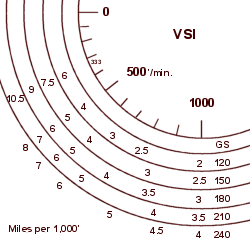Mustang II
Flight Tips
Descent Calculation

Here is a tool that might be of help or interest for calculating descents.
It depicts a "multiplier" to determine how many miles per thousand feet of descent are necessary, depending on your descent rate and groundspeed. Think of it as "Miles per Thousand".

The first image is "full size", the second being small enough to have on your kneeboard or in your "Trip book".
Large image of descent "multipliers"
Small image of descent "multipliers"How it works

Here's what I do, and you can adapt to suit your own airplane(s).
I descend at 75% power and let the speed build up. You will have to adjust Manifold Pressure or RPM and enrichen the mixture as you descend to keep 75% power set. Speed will build up typically 30 knots or so. For passengers with sensitive ears, you may need to descend as slow as 333' per minute, but usually 5-700' per minute is OK if folks can clear their ears OK. Descending at a higher rate is actually less efficient, in my opinion.

Plan to arrive at pattern altitude about 5-10 miles before the airport (depending on airplane type) to allow for deceleration to approach speed and pattern entry. Take your cruise altitude, subtract pattern altitude for altitude to lose. Before descent, and as you're descending, recalculate to see "how it's going" by multiplying "thousands to descend times the multiplier, then add the miles for deceleration. This last number will change depending on whether you're going straight in or doing a full pattern, etc.

Here's an example:

Cruising at 7,500'; airport pattern altitude=1,500'. (Six thousand to descend). Planning to descend at 500' per minute with a 180 knot groundspeed. (Multiplier=6). Planned deceleration mileage at the bottom of the descent: 7 miles.

Start descent: at 43 miles: (6 [thousands] x 6 [multiplier]) + 7
Descending through 5,500' check distance should be 31 miles (4 x 6) + 7
Descending through 2,500' check distance should be 13 miles (1 x 6) + 7.
Bottom of descent at 1,500' should be at 7 miles to start deceleration.

Some notes:
- Note that the "multipliers" are not linear - that is, the 750' / minute multiplier is NOT half way between the 500' and 1000' multipliers. (It's a little less).
- Turbulence will usually require slower speed descents at a lower power setting (at a lower groundspeed), thus maybe a different multiplier.
- In my Mustang II, I usually descend at approximately 6-700' / minute with a groundspeed of approximately 190 to 200 knots, so I use a multiplier of 5, but with a headwind it'll be about 4, and with a nice tailwind, 6.
- The chart is derived by:
Rate of descent= ((Groundspeed / 60) x 1000) / Multiplier
or: Multiplier= (Groundspeed / 60) x (1000 / Rate of descent)

For what it's worth, most jets use approximately a "3 to 1" and then add about 15 miles, depending on several other variables. So for a descent from FL 350 we can go to idle power 120 miles out ((35 x 3) + 15) for the most efficient descent.

Hopefully this offers one way to plan an efficient descent.

Return to ExperimentalAirplane.com